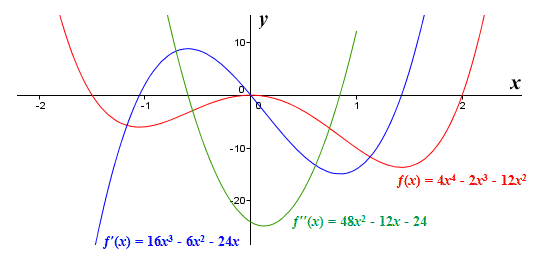top of page

# Invaluable High School Calculus Study Aid!

During the first half of most high school calculus classes, teachers usually hand out some sort of chart like the one seen below:Many students who seek calculus tutoring have a version of this chart, but they don't actually know how to use it. That's a bummer because it is an invaluable tool for many problems kids see on exams, especially in AP calculus.

I like this version above because it has a user-friendly "if/then" structure to help students choose the correct note for the problem they are working on. If they can correctly identify the starting point for the problem at hand (the "if" statement) they can use the chart to find a helpful conclusion (the "then" part).

For example, the top if/then statement on the left side of the chart is the basic calc fact needed to solve many of the problems students will likely see in both 1st and 2nd semester homework, quizzes and tests. It says if f(x) is increasing, then f '(x) is positive. In other words, if a function is getting greater in value, then its derivative has a positive value. This basic fact helps students answer the following questions (and others):

What are the intervals of increasing and decreasing for a function?

What can you conclude about f(x) if f '(x) is positive?

What can you conclude about the motion of a particle if the derivative of its position function is positive?

To increase the effectiveness of this chart, students should, whenever allowed, use graphing calculators and online graphing websites like DESMOS to help visualize the information the chart above is telling you. All of the information in the chart above can also be thought of as hints for making accurate graphs of functions, derivatives and second derivatives.

For example, look at the graph below. Look at the red graph. It is the original function. The red curve starts to swoop upward and increase at about x = -1. When this happens the blue curve rises above the x axis and takes on positive values. When the red curve starts to decrease at x = 0 the blue curve then takes on negative values. This relationship between f (x) and f ' (x) is outlined by the chart above: "if f(x) is increasing, then f '(x) is positive"Try to make more connections between the chart above and this graph. One can find maximums, minimums, intervals of upward and downward concavity and more.

Overall, it's a great math tool for high school calc as well as future calc courses in college!